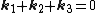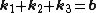# Umklapp process

## Umklapp process

A concept in the theory of transport properties of solids which has to do with the interaction of three or more waves in the solid, such as lattice waves or electron waves. In a continuum, such interactions occur only among waves described by wave vectors k 1, k 2, and so on, such that the interference condition, given by Eq. (1), is satisfied.

(1)The sign of k depends on whether the wave absorbs or emits energy. Since &planck; k is the momentum of a quantum (or particle) described by the wave, Eq. (1) corresponds to conservation of momentum. In a crystal lattice further interactions
(2)occur, satisfying Eq. (2), where b is any integral combination of the three inverse lattice vectors b i, defined by a · b j = 2&pgr;δij, the a 's being the periodicity vectors. The group of processes described in Eq. (2) are the Umklapp processes or flip-over processes, so called because the total momentum of the initial particles or quanta is reversed. See Crystal

McGraw-Hill Concise Encyclopedia of Physics. © 2002 by The McGraw-Hill Companies, Inc.

## Umklapp process

[′u̇m‚kläp ‚prä·səs]
(solid-state physics)
The interaction of three or more waves in a solid, such as lattice waves or electron waves, in which the sum of the wave vectors is not equal to zero but, rather, is equal to a vector in the reciprocal lattice. Also known as flip-over process.
McGraw-Hill Dictionary of Scientific & Technical Terms, 6E, Copyright © 2003 by The McGraw-Hill Companies, Inc.
References in periodicals archive ?
The negative flow expressed with the satisfies the condition of an umklapp process,
The dispersion (the dotted line) in Figure 2(b) evidences the umklapp process. The excitations through the umklapp process result in the in-plane backward shift of rays as depicted in Figure 7.

Site: Follow: Share:
Open / Close